### Automatic Differentiation

PROC OPTMODEL automatically generates statements to evaluate the derivatives for most objective expressions and nonlinear constraints. PROC OPTMODEL generates analytic derivatives for objective and constraint expressions written in terms of the procedure’s mathematical operators and most standard SAS library functions.

Note: Some functions, such as ABS, FLOOR, and SIGN, and some operators, such as IF-THEN, <> (element minimum operator), and >< (element maximum operator), must be used carefully in modeling expressions because functions including such components are not continuously differentiable or even continuous.

Expressions that reference user-defined functions or some SAS library functions might require numerical approximation of derivatives. PROC OPTMODEL uses either forward-difference approximation or central-difference approximation as specified by the FD= option (see the section PROC OPTMODEL Statement).

Note: The numerical gradient approximations are significantly slower than automatically generated derivatives when the number of optimization variables is large.

#### Forward-Difference Approximations

The FD=FORWARD option requests the use of forward-difference derivative approximations. For a function
ofvariables, the first-order derivatives are approximated by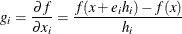Notice that up toadditional function calls are needed here. The step lengths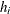,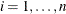, are based on the assumed function precision, DIGITS: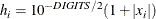You can use the FDIGITS= option to specify the function precision, DIGITS, for the objective function. For constraints, use the CDIGITS= option.

The second-order derivatives are approximated by using up to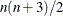extra function calls (Dennis and Schnabel; 1983, pp. 80, 104):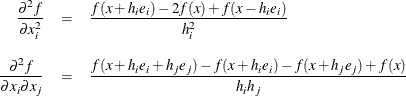Notice that the diagonal of the Hessian uses a central-difference approximation (Abramowitz and Stegun; 1972, p. 884). The step lengths are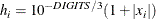#### Central-Difference Approximations

The FD=CENTRAL option requests the use of central-difference derivative approximations. Generally, central-difference approximations are more accurate than forward-difference approximations, but they require more function evaluations. For a functionofvariables, the first-order derivatives are approximated by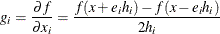Notice that up to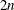additional function calls are needed here. The step lengths,, are based on the assumed function precision, DIGITS:You can use the FDIGITS= option to specify the function precision, DIGITS, for the objective function. For constraints, use the CDIGITS= option.

The second-order derivatives are approximated by using up to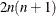extra function calls (Abramowitz and Stegun; 1972, p. 884):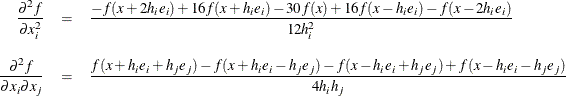The step lengths are